Intermediate Algebra 2e

# 2.1Use a General Strategy to Solve Linear Equations

Intermediate Algebra 2e2.1 Use a General Strategy to Solve Linear Equations

### Learning Objectives

By the end of this section, you will be able to:

• Solve linear equations using a general strategy
• Classify equations
• Solve equations with fraction or decimal coefficients

### Be Prepared 2.1

Before you get started, take this readiness quiz.

Simplify: $32(12x+20).32(12x+20).$
If you missed this problem, review Example 1.51.

### Be Prepared 2.2

Simplify: $5−2(n+1).5−2(n+1).$
If you missed this problem, review Example 1.55.

### Be Prepared 2.3

Find the LCD of $5656$ and $14.14.$
If you missed this problem, review Example 1.28.

### Solve Linear Equations Using a General Strategy

Solving an equation is like discovering the answer to a puzzle. The purpose in solving an equation is to find the value or values of the variable that makes it a true statement. Any value of the variable that makes the equation true is called a solution to the equation. It is the answer to the puzzle!

### Solution of an Equation

A solution of an equation is a value of a variable that makes a true statement when substituted into the equation.

To determine whether a number is a solution to an equation, we substitute the value for the variable in the equation. If the resulting equation is a true statement, then the number is a solution of the equation.

### How To

#### Determine Whether a Number is a Solution to an Equation.

1. Step 1. Substitute the number for the variable in the equation.
2. Step 2. Simplify the expressions on both sides of the equation.
3. Step 3.
Determine whether the resulting equation is true.
• If it is true, the number is a solution.
• If it is not true, the number is not a solution.

### Example 2.1

Determine whether the values are solutions to the equation: $5y+3=10y−4.5y+3=10y−4.$

$y=35y=35$ $y=75y=75$

### Try It 2.1

Determine whether the values are solutions to the equation: $9y+2=6y+3.9y+2=6y+3.$

$y=43y=43$ $y=13y=13$

### Try It 2.2

Determine whether the values are solutions to the equation: $4x−2=2x+1.4x−2=2x+1.$

$x=32x=32$ $x=−12x=−12$

There are many types of equations that we will learn to solve. In this section we will focus on a linear equation.

### Linear Equation

A linear equation is an equation in one variable that can be written, where a and b are real numbers and $a≠0,a≠0,$ as:

$ax+b=0ax+b=0$

To solve a linear equation it is a good idea to have an overall strategy that can be used to solve any linear equation. In the next example, we will give the steps of a general strategy for solving any linear equation. Simplifying each side of the equation as much as possible first makes the rest of the steps easier.

### Example 2.2

#### How to Solve a Linear Equation Using a General Strategy

Solve: $7(n−3)−8=−157(n−3)−8=−15$.

### Try It 2.3

Solve: $2(m−4)+3=−1.2(m−4)+3=−1.$

### Try It 2.4

Solve: $5(a−3)+5=−10.5(a−3)+5=−10.$

These steps are summarized in the General Strategy for Solving Linear Equations below.

### How To

#### Solve linear equations using a general strategy.

1. Step 1. Simplify each side of the equation as much as possible.
Use the Distributive Property to remove any parentheses.
Combine like terms.
2. Step 2. Collect all the variable terms on one side of the equation.
Use the Addition or Subtraction Property of Equality.
3. Step 3. Collect all the constant terms on the other side of the equation.
Use the Addition or Subtraction Property of Equality.
4. Step 4. Make the coefficient of the variable term equal to 1.
Use the Multiplication or Division Property of Equality.
State the solution to the equation.
5. Step 5. Check the solution.
Substitute the solution into the original equation to make sure the result is a true statement.

### Example 2.3

Solve: $23(3m−6)=5−m.23(3m−6)=5−m.$

### Try It 2.5

Solve: $13(6u+3)=7−u.13(6u+3)=7−u.$

### Try It 2.6

Solve: $23(9x−12)=8+2x.23(9x−12)=8+2x.$

We can solve equations by getting all the variable terms to either side of the equal sign. By collecting the variable terms on the side where the coefficient of the variable is larger, we avoid working with some negatives. This will be a good strategy when we solve inequalities later in this chapter. It also helps us prevent errors with negatives.

### Example 2.4

Solve: $4(x−1)−2=5(2x+3)+6.4(x−1)−2=5(2x+3)+6.$

### Try It 2.7

Solve: $6(p−3)−7=5(4p+3)−12.6(p−3)−7=5(4p+3)−12.$

### Try It 2.8

Solve: $8(q+1)−5=3(2q−4)−1.8(q+1)−5=3(2q−4)−1.$

### Example 2.5

Solve: $10[3−8(2s−5)]=15(40−5s).10[3−8(2s−5)]=15(40−5s).$

### Try It 2.9

Solve: $6[4−2(7y−1)]=8(13−8y).6[4−2(7y−1)]=8(13−8y).$

### Try It 2.10

Solve: $12[1−5(4z−1)]=3(24+11z).12[1−5(4z−1)]=3(24+11z).$

### Classify Equations

Whether or not an equation is true depends on the value of the variable. The equation $7x+8=−137x+8=−13$ is true when we replace the variable, x, with the value $−3,−3,$ but not true when we replace x with any other value. An equation like this is called a conditional equation. All the equations we have solved so far are conditional equations.

### Conditional Equation

An equation that is true for one or more values of the variable and false for all other values of the variable is a conditional equation.

Now let’s consider the equation $7y+14=7(y+2).7y+14=7(y+2).$ Do you recognize that the left side and the right side are equivalent? Let’s see what happens when we solve for y.

Solve: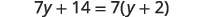Distribute.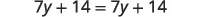Subtract $7y7y$ to each side to get the $y’sy’s$ to one side.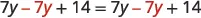Simplify—the y’s are eliminated.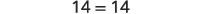But $14=1414=14$ is true.

This means that the equation $7y+14=7(y+2)7y+14=7(y+2)$ is true for any value of y. We say the solution to the equation is all of the real numbers. An equation that is true for any value of the variable is called an identity.

### Identity

An equation that is true for any value of the variable is called an identity.

The solution of an identity is all real numbers.

What happens when we solve the equation $−8z=−8z+9?−8z=−8z+9?$

Solve: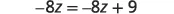Add $8z8z$ to both sides to leave the constant alone on the right.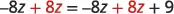Simplify—the $z’sz’s$ are eliminated.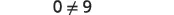But $0≠9.0≠9.$

Solving the equation $−8z=−8z+9−8z=−8z+9$ led to the false statement $0=9.0=9.$ The equation $−8z=−8z+9−8z=−8z+9$ will not be true for any value of z. It has no solution. An equation that has no solution, or that is false for all values of the variable, is called a contradiction.

An equation that is false for all values of the variable is called a contradiction.

The next few examples will ask us to classify an equation as conditional, an identity, or as a contradiction.

### Example 2.6

Classify the equation as a conditional equation, an identity, or a contradiction and then state the solution: $6(2n−1)+3=2n−8+5(2n+1).6(2n−1)+3=2n−8+5(2n+1).$

### Try It 2.11

Classify the equation as a conditional equation, an identity, or a contradiction and then state the solution: $4+9(3x−7)=−42x−13+23(3x−2).4+9(3x−7)=−42x−13+23(3x−2).$

### Try It 2.12

Classify the equation as a conditional equation, an identity, or a contradiction and then state the solution: $8(1−3x)+15(2x+7)=2(x+50)+4(x+3)+1.8(1−3x)+15(2x+7)=2(x+50)+4(x+3)+1.$

### Example 2.7

Classify the equation as a conditional equation, an identity, or a contradiction and then state the solution: $8+3(a−4)=0.8+3(a−4)=0.$

### Try It 2.13

Classify the equation as a conditional equation, an identity, or a contradiction and then state the solution: $11(q+3)−5=19.11(q+3)−5=19.$

### Try It 2.14

Classify the equation as a conditional equation, an identity, or a contradiction and then state the solution: $6+14(k−8)=95.6+14(k−8)=95.$

### Example 2.8

Classify the equation as a conditional equation, an identity, or a contradiction and then state the solution: $5m+3(9+3m)=2(7m−11).5m+3(9+3m)=2(7m−11).$

### Try It 2.15

Classify the equation as a conditional equation, an identity, or a contradiction and then state the solution: $12c+5(5+3c)=3(9c−4).12c+5(5+3c)=3(9c−4).$

### Try It 2.16

Classify the equation as a conditional equation, an identity, or a contradiction and then state the solution: $4(7d+18)=13(3d−2)−11d.4(7d+18)=13(3d−2)−11d.$

We summarize the methods for classifying equations in the table.

Type of equation What happens when you solve it? Solution
Conditional Equation True for one or more values of the variables and false for all other values One or more values
Identity True for any value of the variable All real numbers
Contradiction False for all values of the variable No solution
Table 2.1

### Solve Equations with Fraction or Decimal Coefficients

We could use the General Strategy to solve the next example. This method would work fine, but many students do not feel very confident when they see all those fractions. So, we are going to show an alternate method to solve equations with fractions. This alternate method eliminates the fractions.

We will apply the Multiplication Property of Equality and multiply both sides of an equation by the least common denominator (LCD) of all the fractions in the equation. The result of this operation will be a new equation, equivalent to the first, but without fractions. This process is called clearing the equation of fractions.

To clear an equation of decimals, we think of all the decimals in their fraction form and then find the LCD of those denominators.

### Example 2.9

#### How to Solve Equations with Fraction or Decimal Coefficients

Solve: $112x+56=34.112x+56=34.$

### Try It 2.17

Solve: $14x+12=58.14x+12=58.$

### Try It 2.18

Solve: $18x+12=14.18x+12=14.$

Notice in the previous example, once we cleared the equation of fractions, the equation was like those we solved earlier in this chapter. We changed the problem to one we already knew how to solve. We then used the General Strategy for Solving Linear Equations.

### How To

#### Solve Equations with Fraction or Decimal Coefficients.

1. Step 1. Find the least common denominator (LCD) of all the fractions and decimals (in fraction form) in the equation.
2. Step 2. Multiply both sides of the equation by that LCD. This clears the fractions and decimals.
3. Step 3. Solve using the General Strategy for Solving Linear Equations.

### Example 2.10

Solve: $5=12y+23y−34y.5=12y+23y−34y.$

### Try It 2.19

Solve: $7=12x+34x−23x.7=12x+34x−23x.$

### Try It 2.20

Solve: $−1=12u+14u−23u.−1=12u+14u−23u.$

In the next example, we’ll distribute before we clear the fractions.

### Example 2.11

Solve: $12(y−5)=14(y−1).12(y−5)=14(y−1).$

### Try It 2.21

Solve: $15(n+3)=14(n+2).15(n+3)=14(n+2).$

### Try It 2.22

Solve: $12(m−3)=14(m−7).12(m−3)=14(m−7).$

When you multiply both sides of an equation by the LCD of the fractions, make sure you multiply each term by the LCD—even if it does not contain a fraction.

### Example 2.12

Solve: $4q+32+6=3q+544q+32+6=3q+54$

### Try It 2.23

Solve: $3r+56+1=4r+33.3r+56+1=4r+33.$

### Try It 2.24

Solve: $2s+32+1=3s+24.2s+32+1=3s+24.$

Some equations have decimals in them. This kind of equation may occur when we solve problems dealing with money or percentages. But decimals can also be expressed as fractions. For example, $0.7=7100.7=710$ and $0.29=29100.0.29=29100.$ So, with an equation with decimals, we can use the same method we used to clear fractions—multiply both sides of the equation by the least common denominator.

The next example uses an equation that is typical of the ones we will see in the money applications in a later section. Notice that we will clear all decimals by multiplying by the LCD of their fraction form.

### Example 2.13

Solve: $0.25x+0.05(x+3)=2.85.0.25x+0.05(x+3)=2.85.$

### Try It 2.25

Solve: $0.25n+0.05(n+5)=2.95.0.25n+0.05(n+5)=2.95.$

### Try It 2.26

Solve: $0.10d+0.05(d−5)=2.15.0.10d+0.05(d−5)=2.15.$

### Section 2.1 Exercises

#### Practice Makes Perfect

Solve Equations Using the General Strategy

In the following exercises, determine whether the given values are solutions to the equation.

1.

$6 y + 10 = 12 y 6 y + 10 = 12 y$

$y=53y=53$ $y=−12y=−12$

2.

$4 x + 9 = 8 x 4 x + 9 = 8 x$

$x=−78x=−78$ $x=94x=94$

3.

$8 u − 1 = 6 u 8 u − 1 = 6 u$

$u=−12u=−12$ $u=12u=12$

4.

$9 v − 2 = 3 v 9 v − 2 = 3 v$

$v=−13v=−13$ $v=13v=13$

In the following exercises, solve each linear equation.

5.

$15 ( y − 9 ) = −60 15 ( y − 9 ) = −60$

6.

$−16 ( 3 n + 4 ) = 32 −16 ( 3 n + 4 ) = 32$

7.

$− ( w − 12 ) = 30 − ( w − 12 ) = 30$

8.

$− ( t − 19 ) = 28 − ( t − 19 ) = 28$

9.

$51 + 5 ( 4 − q ) = 56 51 + 5 ( 4 − q ) = 56$

10.

$−6 + 6 ( 5 − k ) = 15 −6 + 6 ( 5 − k ) = 15$

11.

$3 ( 10 − 2 x ) + 54 = 0 3 ( 10 − 2 x ) + 54 = 0$

12.

$−2 ( 11 − 7 x ) + 54 = 4 −2 ( 11 − 7 x ) + 54 = 4$

13.

$2 3 ( 9 c − 3 ) = 22 2 3 ( 9 c − 3 ) = 22$

14.

$3 5 ( 10 x − 5 ) = 27 3 5 ( 10 x − 5 ) = 27$

15.

$1 5 ( 15 c + 10 ) = c + 7 1 5 ( 15 c + 10 ) = c + 7$

16.

$1 4 ( 20 d + 12 ) = d + 7 1 4 ( 20 d + 12 ) = d + 7$

17.

$3 ( 4 n − 1 ) − 2 = 8 n + 3 3 ( 4 n − 1 ) − 2 = 8 n + 3$

18.

$9 ( 2 m − 3 ) − 8 = 4 m + 7 9 ( 2 m − 3 ) − 8 = 4 m + 7$

19.

$12 + 2 ( 5 − 3 y ) = −9 ( y − 1 ) − 2 12 + 2 ( 5 − 3 y ) = −9 ( y − 1 ) − 2$

20.

$−15 + 4 ( 2 − 5 y ) = −7 ( y − 4 ) + 4 −15 + 4 ( 2 − 5 y ) = −7 ( y − 4 ) + 4$

21.

$5 + 6 ( 3 s − 5 ) = −3 + 2 ( 8 s − 1 ) 5 + 6 ( 3 s − 5 ) = −3 + 2 ( 8 s − 1 )$

22.

$−12 + 8 ( x − 5 ) = −4 + 3 ( 5 x − 2 ) −12 + 8 ( x − 5 ) = −4 + 3 ( 5 x − 2 )$

23.

$4 ( p − 4 ) − ( p + 7 ) = 5 ( p − 3 ) 4 ( p − 4 ) − ( p + 7 ) = 5 ( p − 3 )$

24.

$3 ( a − 2 ) − ( a + 6 ) = 4 ( a − 1 ) 3 ( a − 2 ) − ( a + 6 ) = 4 ( a − 1 )$

25.

$4 [ 5 − 8 ( 4 c − 3 ) ] = 12 ( 1 − 13 c ) − 8 4 [ 5 − 8 ( 4 c − 3 ) ] = 12 ( 1 − 13 c ) − 8$

26.

$5 [ 9 − 2 ( 6 d − 1 ) ] = 11 ( 4 − 10 d ) − 139 5 [ 9 − 2 ( 6 d − 1 ) ] = 11 ( 4 − 10 d ) − 139$

27.

$3 [ −9 + 8 ( 4 h − 3 ) ] = 2 ( 5 − 12 h ) − 19 3 [ −9 + 8 ( 4 h − 3 ) ] = 2 ( 5 − 12 h ) − 19$

28.

$3 [ −14 + 2 ( 15 k − 6 ) ] = 8 ( 3 − 5 k ) − 24 3 [ −14 + 2 ( 15 k − 6 ) ] = 8 ( 3 − 5 k ) − 24$

29.

$5 [ 2 ( m + 4 ) + 8 ( m − 7 ) ] = 2 [ 3 ( 5 + m ) − ( 21 − 3 m ) ] 5 [ 2 ( m + 4 ) + 8 ( m − 7 ) ] = 2 [ 3 ( 5 + m ) − ( 21 − 3 m ) ]$

30.

$10 [ 5 ( n + 1 ) + 4 ( n − 1 ) ] = 11 [ 7 ( 5 + n ) − ( 25 − 3 n ) ] 10 [ 5 ( n + 1 ) + 4 ( n − 1 ) ] = 11 [ 7 ( 5 + n ) − ( 25 − 3 n ) ]$

Classify Equations

In the following exercises, classify each equation as a conditional equation, an identity, or a contradiction and then state the solution.

31.

$23 z + 19 = 3 ( 5 z − 9 ) + 8 z + 46 23 z + 19 = 3 ( 5 z − 9 ) + 8 z + 46$

32.

$15 y + 32 = 2 ( 10 y − 7 ) − 5 y + 46 15 y + 32 = 2 ( 10 y − 7 ) − 5 y + 46$

33.

$18 ( 5 j − 1 ) + 29 = 47 18 ( 5 j − 1 ) + 29 = 47$

34.

$24 ( 3 d − 4 ) + 100 = 52 24 ( 3 d − 4 ) + 100 = 52$

35.

$22 ( 3 m − 4 ) = 8 ( 2 m + 9 ) 22 ( 3 m − 4 ) = 8 ( 2 m + 9 )$

36.

$30 ( 2 n − 1 ) = 5 ( 10 n + 8 ) 30 ( 2 n − 1 ) = 5 ( 10 n + 8 )$

37.

$7 v + 42 = 11 ( 3 v + 8 ) − 2 ( 13 v − 1 ) 7 v + 42 = 11 ( 3 v + 8 ) − 2 ( 13 v − 1 )$

38.

$18 u − 51 = 9 ( 4 u + 5 ) − 6 ( 3 u − 10 ) 18 u − 51 = 9 ( 4 u + 5 ) − 6 ( 3 u − 10 )$

39.

$45 ( 3 y − 2 ) = 9 ( 15 y − 6 ) 45 ( 3 y − 2 ) = 9 ( 15 y − 6 )$

40.

$60 ( 2 x − 1 ) = 15 ( 8 x + 5 ) 60 ( 2 x − 1 ) = 15 ( 8 x + 5 )$

41.

$9 ( 14 d + 9 ) + 4 d = 13 ( 10 d + 6 ) + 3 9 ( 14 d + 9 ) + 4 d = 13 ( 10 d + 6 ) + 3$

42.

$11 ( 8 c + 5 ) − 8 c = 2 ( 40 c + 25 ) + 5 11 ( 8 c + 5 ) − 8 c = 2 ( 40 c + 25 ) + 5$

Solve Equations with Fraction or Decimal Coefficients

In the following exercises, solve each equation with fraction coefficients.

43.

$1 4 x − 1 2 = − 3 4 1 4 x − 1 2 = − 3 4$

44.

$3 4 x − 1 2 = 1 4 3 4 x − 1 2 = 1 4$

45.

$5 6 y − 2 3 = − 3 2 5 6 y − 2 3 = − 3 2$

46.

$5 6 y − 1 3 = − 7 6 5 6 y − 1 3 = − 7 6$

47.

$1 2 a + 3 8 = 3 4 1 2 a + 3 8 = 3 4$

48.

$5 8 b + 1 2 = − 3 4 5 8 b + 1 2 = − 3 4$

49.

$2 = 1 3 x − 1 2 x + 2 3 x 2 = 1 3 x − 1 2 x + 2 3 x$

50.

$2 = 3 5 x − 1 3 x + 2 5 x 2 = 3 5 x − 1 3 x + 2 5 x$

51.

$1 3 w + 5 4 = w − 1 4 1 3 w + 5 4 = w − 1 4$

52.

$1 2 a − 1 4 = 1 6 a + 1 12 1 2 a − 1 4 = 1 6 a + 1 12$

53.

$1 3 b + 1 5 = 2 5 b − 3 5 1 3 b + 1 5 = 2 5 b − 3 5$

54.

$1 3 x + 2 5 = 1 5 x − 2 5 1 3 x + 2 5 = 1 5 x − 2 5$

55.

$1 4 ( p − 7 ) = 1 3 ( p + 5 ) 1 4 ( p − 7 ) = 1 3 ( p + 5 )$

56.

$1 5 ( q + 3 ) = 1 2 ( q − 3 ) 1 5 ( q + 3 ) = 1 2 ( q − 3 )$

57.

$1 2 ( x + 4 ) = 3 4 1 2 ( x + 4 ) = 3 4$

58.

$1 3 ( x + 5 ) = 5 6 1 3 ( x + 5 ) = 5 6$

59.

$4 n + 8 4 = n 3 4 n + 8 4 = n 3$

60.

$3 p + 6 3 = p 2 3 p + 6 3 = p 2$

61.

$3 x + 4 2 + 1 = 5 x + 10 8 3 x + 4 2 + 1 = 5 x + 10 8$

62.

$10 y − 2 3 + 3 = 10 y + 1 9 10 y − 2 3 + 3 = 10 y + 1 9$

63.

$7 u − 1 4 − 1 = 4 u + 8 5 7 u − 1 4 − 1 = 4 u + 8 5$

64.

$3 v − 6 2 + 5 = 11 v − 4 5 3 v − 6 2 + 5 = 11 v − 4 5$

In the following exercises, solve each equation with decimal coefficients.

65.

$0.4 x + 0.6 = 0.5 x − 1.2 0.4 x + 0.6 = 0.5 x − 1.2$

66.

$0.7 x + 0.4 = 0.6 x + 2.4 0.7 x + 0.4 = 0.6 x + 2.4$

67.

$0.9 x − 1.25 = 0.75 x + 1.75 0.9 x − 1.25 = 0.75 x + 1.75$

68.

$1.2 x − 0.91 = 0.8 x + 2.29 1.2 x − 0.91 = 0.8 x + 2.29$

69.

$0.05 n + 0.10 ( n + 8 ) = 2.15 0.05 n + 0.10 ( n + 8 ) = 2.15$

70.

$0.05 n + 0.10 ( n + 7 ) = 3.55 0.05 n + 0.10 ( n + 7 ) = 3.55$

71.

$0.10 d + 0.25 ( d + 5 ) = 4.05 0.10 d + 0.25 ( d + 5 ) = 4.05$

72.

$0.10 d + 0.25 ( d + 7 ) = 5.25 0.10 d + 0.25 ( d + 7 ) = 5.25$

#### Everyday Math

73.

Fencing Micah has 74 feet of fencing to make a dog run in his yard. He wants the length to be 2.5 feet more than the width. Find the length, L, by solving the equation $2L+2(L−2.5)=74.2L+2(L−2.5)=74.$

74.

Stamps Paula bought \$22.82 worth of 49-cent stamps and 21-cent stamps. The number of 21-cent stamps was eight less than the number of
49-cent stamps. Solve the equation
$0.49s+0.21​(s−8)​​=22.820.49s+0.21​(s−8)​​=22.82$ for s, to find the number of 49-cent stamps Paula bought.

#### Writing Exercises

75.

Using your own words, list the steps in the general strategy for solving linear equations.

76.

Explain why you should simplify both sides of an equation as much as possible before collecting the variable terms to one side and the constant terms to the other side.

77.

What is the first step you take when solving the equation $3−7(y−4)=38?3−7(y−4)=38?$ Why is this your first step?

78.

If an equation has several fractions, how does multiplying both sides by the LCD make it easier to solve?

79.

If an equation has fractions only on one side, why do you have to multiply both sides of the equation by the LCD?

80.

For the equation $0.35x+2.1=3.85,0.35x+2.1=3.85,$ how do you clear the decimal?

#### Self Check

After completing the exercises, use this checklist to evaluate your mastery of the objectives of this section.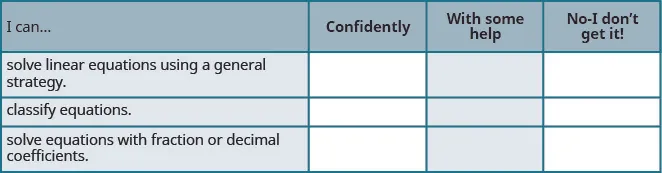If most of your checks were:

…confidently. Congratulations! You have achieved the objectives in this section. Reflect on the study skills you used so that you can continue to use them. What did you do to become confident of your ability to do these things? Be specific.

…with some help. This must be addressed quickly because topics you do not master become potholes in your road to success. In math every topic builds upon previous work. It is important to make sure you have a strong foundation before you move on. Whom can you ask for help?Your fellow classmates and instructor are good resources. Is there a place on campus where math tutors are available? Can your study skills be improved?

…no - I don’t get it! This is a warning sign and you must not ignore it. You should get help right away or you will quickly be overwhelmed. See your instructor as soon as you can to discuss your situation. Together you can come up with a plan to get you the help you need.

Order a print copy

As an Amazon Associate we earn from qualifying purchases.